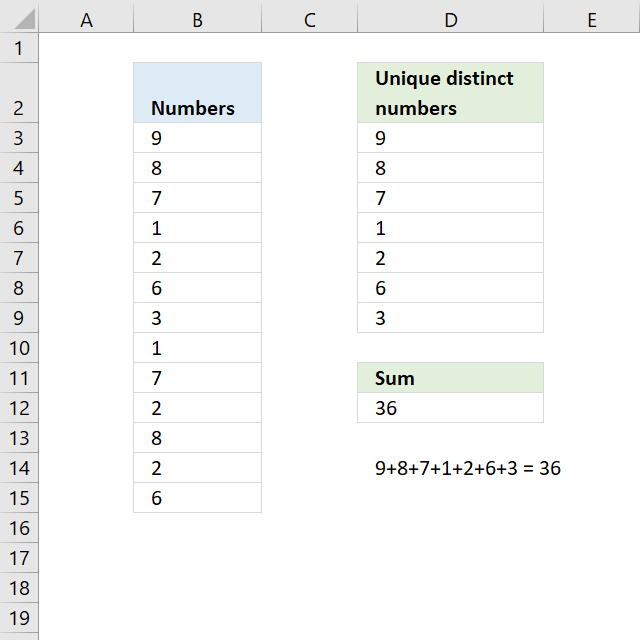Author: Oscar Cronquist Article last updated on November 16, 2018The image above shows numbers in column B, some of these numbers are duplicates. The formula in D12 adds unique distinct numbers meaning the first instance of each number is added to the total, however, the duplicates are ignored.

=SUMPRODUCT((1/COUNTIF(B3:B15,B3:B15))*B3:B15)

### Explaining formula in cell D12

If you want to examine the formula yourself then go to tab "Formula" on the ribbon and then press with left mouse button on "Evaluate Formula" button.

This will show the calculation steps, one at a time.  Simply press with left mouse button on the Evaluate button to move to next calculation step.

#### Step 1 - Count each value in cell range

The COUNTIF function counts each value in the cell range and returns an array of numbers which shows the number of instances each value has.

COUNTIF(B3:B15,B3:B15)

becomes

COUNTIF({9;8;7;1;2;6;3;1;7;2;8;2;6},{9;8;7;1;2;6;3;1;7;2;8;2;6})

and returns

{1;2;2;2;3;2;1;2;2;3;2;3;2}.

#### Step 2 - Divide 1 with array

If a number has a duplicate then there are two instances of that value. Divide 1 with 2 and we get 0.5, now multiply that value with the number and we get the number to add to the total.

1/COUNTIF(B3:B15,B3:B15)

becomes

1/{1;2;2;2;3;2;1;2;2;3;2;3;2}

and returns

{1;0.5;0.5;0.5;0.333333333333333;0.5;1;0.5;0.5;0.333333333333333;0.5;0.333333333333333;0.5}

#### Step 3 - Multiply with numbers

The parentheses make sure that the order of calculation is correct.

(1/COUNTIF(B3:B15,B3:B15))*B3:B15

becomes

{1;0.5;0.5;0.5;0.333333333333333;0.5;1;0.5;0.5;0.333333333333333;0.5;0.333333333333333;0.5}*B3:B15

and returns

{9;4;3.5;0.5;0.666666666666667;3;3;0.5;3.5;0.666666666666667;4;0.666666666666667;3}

#### Step 4 - Add numbers

The SUMPRODUCT function is a better option than the SUM function, we don't need to enter the formula as an array formula now.

SUMPRODUCT((1/COUNTIF(B3:B15,B3:B15))*B3:B15)

becomes

SUMPRODUCT({9;4;3.5;0.5;0.666666666666667;3;3;0.5;3.5;0.666666666666667;4;0.666666666666667;3})

and returns 36 in cell D12.

### Get Excel *.xlsx file

Sum unique distinct numbers.xlsx